DownloadDocumentationHNS → Comparative information about HNS

## Comparative information about HNS, expedient for the use in digital filters

The most essential HNS of second, third and fourth dimensions which are expedient to use for digital filters design, isomorphism between them, formulas of linear transformations implementing this isomorphism, are considered in work. Comparative information on the amount of executable operations with real numbers on 1 multiplication of hypercomplex numbers is given.

1. Systems of dimension 2.
A) System of complex numbers.
 e1 e2 e1 e1 e2 e2 e2 -e1

Multiplication of two numbersrequires 4 multiplications and 2 additions of real numbers.
Use of formula:requires 3 multiplications and 5 additions.

B) System of double numbers.
 e1 e2 e1 e1 e2 e2 e2 e1

Multiplication of two numbers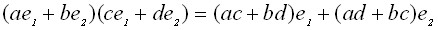requires 4 multiplications and 2 additions of real numbers.

It is expedient to move to isomorphic system with multiplication table:
 E1 E2 E1 E1 0 E2 0 E2

where multiplication is carried out by formula: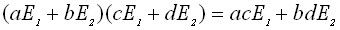Isomorphism between these systems is defined by formulae: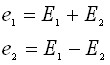Thus, multiplication with the use of this system, even taking into account direct and reverse transitions, requires only 2 multiplications on real numbers, and 2 additions.

2. Systems of dimension 3.

Isomorphism between the system of triplex numbers of Lyusha T and direct sum of real and complex numberswith multiplication tables has the following form:
 e1 e2 e3 E1 E2 E3 e1 e1 e2 e3 E1 E1 0 0 e2 e2 (e3 – e2)/2 –e2 E2 0 E2 E3 e3 e3 –e2 e1 E3 0 E3 –E2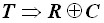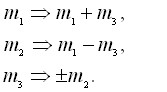Evidently, multiplication in the system of triplex numbers of Lyusha T requires 10 multiplications and 1 addition in the system of real numbers, and insystem, taking into account direct and reverse transitions, multiplication requires only 4 multiplications and 7 additions.

3. Systems of dimension 4.

Isomorphism between the systems of quadriplexand bycomplexnumbers with multiplication tables:

 e1 e2 e3 e4 E1 E2 E3 E4 e1 e1 e2 e3 e4 E1 E1 E2 0 0 e2 e2 -e1 e4 - e3 E2 E2 - E1 0 0 e3 e3 e4 - e1 - e2 E3 0 0 E3 E4 e4 e4 - e3 - e2 e1 E4 0 0 E4 - E3

is defined by formulae: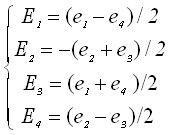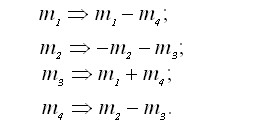Evidently, multiplication in the system of quadriplex numbers requires 16 multiplications and 12 additions in the system of real numbers, and in the system of bycomplex numbers, taking into account direct and reverse transitions, multiplication requires only 6 multiplications and 14 additions.

 ← Previous Next →